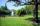# Heron's formula - math problems

The Heron's formula is used to calculate the contents of a general triangle using the lengths of its sides. Heron's formula states that the area of a triangle whose sides have lengths a, b, and c is:
$S=\sqrt{s\left(s-a\right)\left(s-b\right)\left(s-c\right)}$
, where
$s=\frac{a+b+c}{2}$
is the semiperimeter (half perimeter) of the triangle .

#### Number of problems found: 40

• Sides of the triangleCalculate triangle sides where its area is S = 84 cm2 and a = x, b = x + 1, xc = x + 2
• TriangleDetermine whether we can make a triangle with the given side lengths. If so, use Heron's formula to find the area of the triangle. a = 158 b = 185 c = 201
• Find the areaFind the area of the triangle with the given measurements. Round the solution to the nearest hundredth if necessary. A = 50°, b = 30 ft, c = 14 ft
• The farmerThe farmer would like to first seed his small field. The required amount depends on the seed area. Field has a triangular shape. The farmer had fenced field, so he knows the lengths of the sides: 119, 111 and 90 meters. Find a suitable way to determine th
• Cardboard boxWe want to make a cardboard box shaped quadrangular prism with rhombic base. Rhombus has a side of 5 cm and 8 cm one diagonal long. The height of the box to be 12 cm. The box will be open at the top. How many square centimeters cardboard we need, if we ca
• Trapezoid - hard exampleBase of the trapezoid are: 24, 16 cm. Diagonal 22, 26 cm. Calculate its area and perimeter.
• SailsWe known heights 220, 165, and 132 of sail. It has a triangular shape. What is the surface of the sail?
• ParkIn the newly built park will be permanently placed a rotating sprayer irrigation of lawns. Determine the largest radius of the circle which can irrigate by sprayer P so not to spray park visitors on line AB. Distance AB = 55 m, AP = 36 m and BP = 28 m.
• TriangleThe triangle has known all three sides: a=5.5 m, b=5.3 m, c= 7.8 m. Calculate area of ​this triangle.
• BoxCardboard box-shaped quadrangular prism with a rhombic base. Rhombus has a side 5 cm, and one diagonal 8 cm long, and the box's height is 12 cm. The box will open at the top. How many cm2 of cardboard do we need to cover overlap and joints that are 5% of
• DiamondRhombus has side 17 cm and and one of diagonal 22 cm long. Calculate its area.
• PrismCalculate the volume of the rhombic prism. The prism base is a rhombus whose one diagonal is 47 cm, and the edge of the base is 28 cm. The edge length of the base of the prism and height is 3:5.
• TrianglePlane coordinates of vertices: K[11, -10] L[10, 12] M[1, 3] give Triangle KLM. Calculate its area and its interior angles.
• Triangle ABCCalculate the sides of triangle ABC with area 1404 cm2 and if a: b: c = 12:7:18
• DescribedCalculate perimeter of the circle described by a triangle with sides 478, 255, 352.
• Circles 2Calculate the area bounded by the circumscribed and inscribed circle in triangle with sides 12 cm, 14 cm, 18 cm.
• Heron backlawCalculate missing side in a triangle with sides 17 and 34 and area 275.
• TriangleCalculate heights of the triangle ABC if sides of the triangle are a=82, b=44, and c=53.
• TrapezoidCalculate the area of trapezoid ABCD with sides |AB|= 50 cm, |BC|=25 cm, |CD|=10 cm, |AD|=25 cm..
• Triangle SSSCalculate perimeter and area of ​​a triangle ABC, if a=53, b=46 and c=40.

Do you have an exciting math question or word problem that you can't solve? Ask a question or post a math problem, and we can try to solve it.

We will send a solution to your e-mail address. Solved examples are also published here. Please enter the e-mail correctly and check whether you don't have a full mailbox.